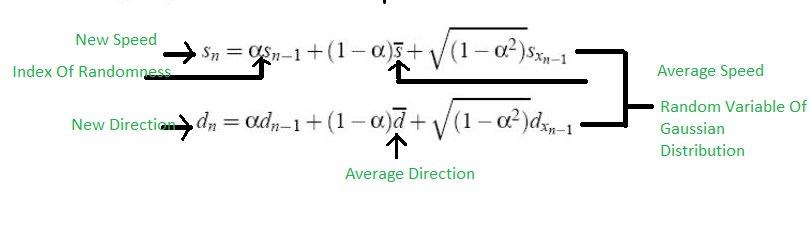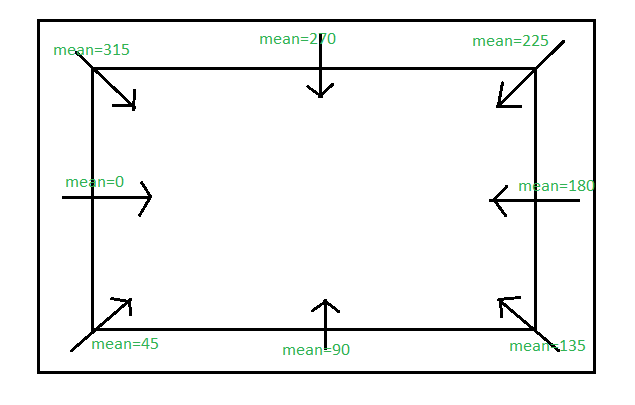Related Articles
Outdoor Mobility Model | Gauss-Markov
• Last Updated : 13 Aug, 2020

There are Indoor Mobility Models like Random-Walk, Random Way-Point, Random Direction. Similarly, there are Outdoor Mobility Models like Gauss Markov and Probabilistic version of Random-Walk.

Gauss-Markov :
This mobility model was proposed for the simulation of the Personal Communication Service. For example, some years ago, there was only landline but now there are wireless phones which you can use by walking all around the house. For that type of service, the Gauss Markov model is used.

The model adapts to different levels of randomness. In indoor mobility models, there are fixed simulation areas in which we can do whether random walk or random way-point or random direction. But in outdoor mobility model, there is no concept of the simulation area. It is purely random.

Initially, each mobile node is assigned a current speed and direction. It means that every node has its initial direction and initial speed and it can change itself randomly. It can move freely in and out.

The value of speed and direction at the nth instance is calculated using the following formula. New speed and New direction can be easily calculated using the formula :Now, as there is no simulation area in this model. Suppose, it goes out of that area or is at the border of the area. Then, the average speed and average direction value are replaced by the mean value. To calculate the mean value we use a protractor. Joining two protractors, we have a full 360 degrees.It looks after the near ranges where it lies. Suppose, it is near the range 225. It covers that in mean and get it inside and gets it full coverage. The main point to look after is only the index of randomness, average speed and random variable of gaussian distribution.

Advantage – It is very simple to understand.
Disadvantage – It degrades the image details and edges of the image.

My Personal Notes arrow_drop_up